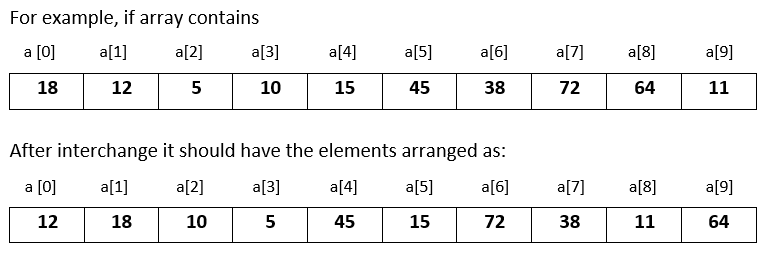# Input 10 numbers in 1d array and interchange the consecutive numbers in C++

###### One Dimensional Array - Question 8

In this question, we will see how to input 10 numbers in a one dimensional integer array and interchange the consecutive numbers in it in C++ programming. To know more about one dimensional array click on the one dimensional array lesson.

Q8) Write a program in C++ to input 10 numbers in a one dimensional integer array and interchange the consecutive numbers in it. That is, interchange a with a, a with a, a with a and so on.#### Program

``````#include <iostream>
#include <conio.h>

using namespace std;

int main()
{
int a, i,t;

cout<<"Enter 10 numbers\n";
for(i=0; i<10; i++)
{
cin>>a[i];
}

//Interchanging the consecutive numbers in array
for(i=0; i<10; i=i+2)
{
t=a[i];
a[i]=a[i+1];
a[i+1]=t;
}

cout<<"\nModified array after interchanging the consecutive numbers\n";
for(i=0; i<10; i++)
{
cout<<a[i]<<" ";
}
return 0;
}``````

#### Output

```Enter 10 numbers
18
12
5
10
15
45
38
72
64
11

Modified array after interchanging the consecutive numbers
12 18 10 5 45 15 72 38 11 64```